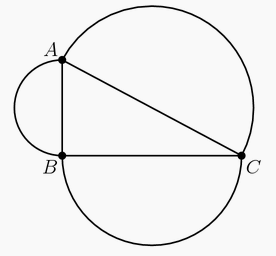Circle AMC8
2013

Problem - 1222
Angle $ABC$ of $\triangle ABC$ is a right angle. The sides of $\triangle ABC$ are the diameters of semicircles as shown. The area of the semicircle on $\overline{AB}$ equals $8\pi$, and the arc of the semicircle on $\overline{AC}$ has length $8.5\pi$. What is the radius of the semicircle on $\overline{BC}$?